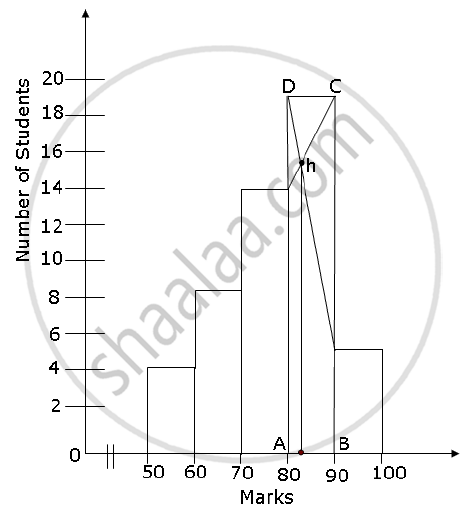Share

# A Mathematics Aptitude Test of 50 Students Was Recorded as Follows: Draw a Histogram from the Above Data Using a Graph Paper and Locate the Mode. - ICSE Class 10 - Mathematics

ConceptGraphical Representation of Histograms

#### Question

A Mathematics aptitude test of 50 students was recorded as follows:

 Marks 50 - 60 60 - 70 70 - 80 80 - 90 90 – 100 No. of Students 4 8 14 19 5

Draw a histogram from the above data using a graph paper and locate the mode.

#### Solution

The histogram for the given data can be drawn by taking the marks on the x-axis and the number of students on the y-axis.To locate the mode from the histogram, we proceed as follows:

1) Find the modal class. Rectangle ABCD is the largest rectangle. It represents the modal class, that is, the mode lies in this rectangle. The modal class is 80 – 90.

2) Draw two lines diagonally from the vertices C and D to the upper corners of the two adjacent rectangles. Let these rectangles intersect at point H.

3) The x-value of the point H is the mode. Thus, mode of the given data is approximately 83.

Is there an error in this question or solution?

#### APPEARS IN

2010-2011 (March) (with solutions)
Question 4.3 | 4.00 marks
Solution A Mathematics Aptitude Test of 50 Students Was Recorded as Follows: Draw a Histogram from the Above Data Using a Graph Paper and Locate the Mode. Concept: Graphical Representation of Histograms.
S